Evaluation of the generalized gamma as a tool for treatment planning optimization

Evaluation of the generalized gamma as a tool for treatment planning optimization
1, 2343322, 3
1Laboratory of Medical Physics, Medical School, University of Patras, Patras, Greece
2Division of Medical Radiation Physics, Karolinska Institutet and Stockholm University, Stockholm, Sweden
3Division of Medical Physics, Department of Radiation Oncology, University of Texas Health Science Center, San Antonio, TX, USA
4Department of Medical Radiological Technologists, Technological Education Institute of Athens, Greece

Cite this article as: Petrou EI, Narayanasamy G, Lavdas E, Stathakis S, Papanikolaou N, Lind BK, Mavroidis P. Evaluation of the generalized gamma as a tool for treatment planning optimization. Int J Cancer Ther Oncol 2014; 2(4):020418.
DOI:
http://dx.doi.org/10.14319/ijcto.0204.18

Original Article

Abstract

Purpose: The aim of that work is to study the theoretical behavior and merits of the Generalized Gamma (generalized dose response gradient) as well as to investigate the usefulness of this concept in practical radiobiological treatment planning.

Methods: In this study, the treatment planning system RayStation 1.9 (Raysearch Laboratories AB, Stockholm, Sweden) was used. Furthermore, radiobiological models that provide the tumor control probability (TCP), normal tissue complication probability (NTCP), complication-free tumor control probability (P+) and the Generalized Gamma were employed. The Generalized Gammas of TCP and NTCP, respectively were calculated for given heterogeneous dose distributions to different organs in order to verify the TCP and NTCP computations of the treatment planning system. In this process, a treatment plan was created, where the target and the organs at risk were included in the same ROI in order to check the validity of the system regarding the objective function P+ and the Generalized Gamma. Subsequently, six additional treatment plans were created with the target organ and the organs at risk placed in the same or different ROIs. In these plans, the mean dose was increased in order to investigate the behavior of dose change on tissue response and on Generalized Gamma before and after the change in dose. By theoretically calculating these quantities, the agreement of different theoretical expressions compared to the values that the treatment planning system provides could be evaluated. Finally, the relative error between the real and approximate response values using the Poisson and the Probit models, for the case of having a target organ consisting of two compartments in a parallel architecture and with the same number of clonogens could be investigated and quantified.

Results: The computations of the RayStation regarding the values of the Generalized Gamma and the objective function (P+) were verified by using an independent software. Furthermore, it was proved that after a small change in dose, the organ that is being affected most is the organ with the highest Generalized Gamma. Apart from that, the validity of the theoretical expressions that describe the change in response and the associated Generalized Gamma was verified but only for the case of small change in dose. Especially for the case of 50% TCP and NTCP, the theoretical values (ΔPapprox.) and those calculated by the RayStation show close agreement, which proves the high importance of the D50 parameter in specifying clinical response levels. Finally, the presented findings show that the behavior of ΔPapprox. looks sensible because, for both of the models that were used (Poisson and Probit), it significantly approaches the real ΔP around the region of 37% and 50% response. The present study managed to evaluate the mathematical expression of Generalized Gamma for the case of non-uniform dose delivery and the accuracy of the RayStation to calculate its values for different organs.

Conclusion: A very important finding of this work is the establishment of the usefulness and clinical relevance of Generalized Gamma. That is because it gives the planner the opportunity to precisely determine which organ will be affected most after a small increase in dose and as a result an optimal treatment plan regarding tumor control and normal tissue complications can be found.

Keywords: Prostate Cancer; Radiobiological Treatment Planning; Generalized Gamma; TCP; NTCP

Introduction

Recent technological developments have introduced dramatic changes in the field of Radiotherapy.1, 2 Radiological imaging has become more advanced providing information at functional level. In this way, a better assessment of the spread, cell density and radiosensitivity variation of clonogenic tumor cells can be accomplished.3 For normal tissues, information on the location and distribution of radiation sensitive functional subunits can be assessed.4, 5 Furthermore, the possibility of calculating now the fractional and through them the composite dose distributions delivered to the patients in a 3-dimensional mode gives a better view of the effectiveness of the applied treatment configurations.6, 7 This abundance of information needs to be accurately used in order to achieve a close agreement between treatment planning and clinical outcome.

Modern treatment planning algorithms try to maximize the conformation of the delivered dose distribution to the target volume through three-dimensional intensity modulated treatment planning, which conforms the high dose region to the shape of the target volume and takes into account the location of the organs at risk (OAR).8-11 Commonly, the irradiation protocols apply dose prescriptions and constraints that have been associated with certain clinically accepted tumor control and normal tissue complication rates. In the current practice, the mean dose in the target volume and additional dose-volume points in the targets and organs at risk are mainly used in treatment plan optimization as objective functions or constraints, respectively.

However, the clinical outcome of a radiotherapy treatment in terms of tumor control and normal tissue complications is nearly always linked to a degree of uncertainty.12, 13 This is partly because two treatment fractions of the same beam configuration are not exactly the same since the nature of radiation beams are stochastic at a microscopic level. Furthermore, the inter-patient and cellular radiosensitivity variations are generally unknown. For these reasons, the expected outcome of a treatment is expressed as the probability of having a certain treatment effect. Radiobiological treatment planning estimates these probabilities for each target and organ at risk of a given clinical case based on the applied dose-distribution and radiobiological data.14-16

Currently, the standard tools that are used for radiotherapy treatment plan evaluation (e.g. isodose distribution, dose volume histogram (DVH), etc) are based on dose only and they do not take the radiobiological characteristics of tumors or normal tissues into account. To cover this gap, the concepts of Tumor Control Probability (TCP), Normal Tissue Complication Probability (NTCP) and complication-free tumor control (P+) were initially introduced to provide an additional plan evaluation analysis.8 More recently, the quantities of the Normalized Dose-response gradient and the Generalized Dose-response gradient were proposed as a supplementary tool in the optimization of treatment plans involving non-uniform dose deliveries, respectively.17

The principle aim of this study is to investigate the theoretical behavior and merits of the Generalized Gamma (generalized dose response gradient) concept. The secondary goal is to examine the usefulness and clinical relevance of the Generalized Gamma in practical radiobiological treatment planning through its implementation in the RayStation® treatment planning system.

Methods and Materials

Definition of the Generalized Gamma
The normalized dose-response gradient was introduced in the 80s (Brahme 1984) and it has extensively been used for describing the dose-response relations of both tumors and normal tissues.18 The normalized dose-response gradient γ(D) can be defined at any dose level D according to the following expression: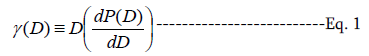where, P(D) is the response of the examined tissue to a given dose, D. The most useful features of γ is that it can be used to predict the change in response from a small change in dose according to the following formula: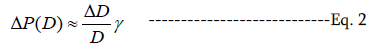From a clinical and radiobiological point of view, it has always been important to know the value of the steepest gradient of the dose-response relationship. This value is denoted as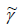and is defined as follows: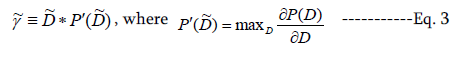In 2001, the concept of the normalized dose-response gradient was generalized to account for non-uniform dose delivery, 17 by explicitly extending the definition of γ as a function of a 3-dimensional dose distribution and by replacing the derivative in Equation (1) with a gradient as shown below: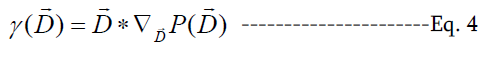This definition makes it possible to calculate the normalized dose-response gradients of different tumors and healthy tissues receiving a given dose distribution and to relate them to various clinical endpoints. The magnitude of the different γ values gives the planner a hint about the modification of the dose distribution needed in order to most effectively decrease normal tissue complications and maximize the probability of complication free cure.

Mathematical formulae related to dose-response gradient
One of the radiobiological models that have extensively been used to describe the dose-response relation of tumors and normal tissues is the linear-quadratic model, which is given by the following mathematical expression: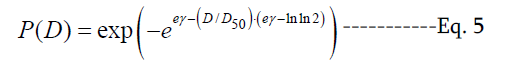where D50, which is the dose that produces a given response to 50% of the patients and γ, which is the maximum normalized gradient of the dose-response curve.4, 15, 19 In order to calculate the normal tissue complication probability (NTCP) of an OAR to a given heterogeneous dose distribution, the relative seriality model was used:4, 15, 20, 21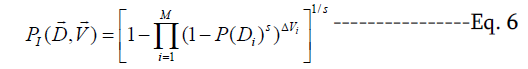where, M is the total number of voxels or subvolumes in the tissue, ΔVi is the fractional irradiated subvolume of an organ compared to the reference volume, Vref for which the values of D50 and γ were calculated and s is the relative seriality parameter that characterizes the internal organization of the organ. P(Di) is the probability of response of the organ having reference volume and being irradiated to dose Di as described by Equation (5).

Regarding the calculation of the TCP it is assumed that the tumor has a parallel structural organization since the eradi-cation of all the clonogenic cells is required. This prerequisite is satisfied by the following relationship:15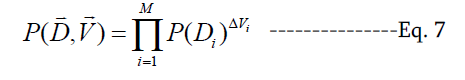Finally, regarding the calculation of the Generalized Gamma, the following relationship was used: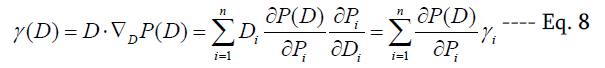where, Di is the quasi-uniform dose in voxel i and γi is the local contribution of voxel i to the Generalized Gamma and it is given by the following equation: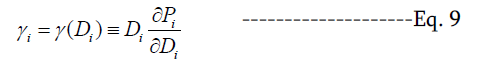Treatment planning specification and optimization by using RayStation
The platform that was used for conducting the present study is RayStation 1.9 (Raysearch Laboratories AB, Sweden). RayStation is among the few treatment planning systems that can produce treatment plans combining different radiation modalities and optimize them using radiobiological measures such as the Generalized Gamma.

In this study, data from prostate cancer cases were used. So, the target of the examined treatment plans was the prostate region and the optimization algorithm calculated the value of the Generalized Gamma for this target while optimizing the plan. During this process, the number of beams, their energy and portals as well as the overall geometry of the treatment configuration were specified. More specifically, the MLC Step and Shoot IMRT radiation modality was used in all the plans. Three 6MV beams were used (gantry angles: 72, 180 and 288 degrees) to achieve an acceptable treatment plan. The dose prescription and number of fractions in every plan were determined by the optimal P+ value, whereas the predetermined dose per fraction was 2.0 Gy. The primary organs at risk (OAR) involved in this case are bladder and rectum. Also, for the different goals of the study, different regions of interest (ROI) were defined. A number of measures were used as evaluators of the quality of the treatment plan such as the minimum dose to the target (Dmin), maximum doses to the OARs (Dmax, which corresponds to D1cc) as well as dose volume histogram (DVH) constraints. Furthermore, radiobiological measures such as the Tumor Control Probability (TCP), the Normal Tissue Compatibility Probability (NTCP) and the complication-free tumor control probability (P+) were employed. The TCP function corresponds to the probability of tumor control, whereas the NTCP function corresponds to the probability of having normal tissue complications, due to radiotherapy. Finally, the objective function P+ corresponds to the probability of achieving tumor control without having any normal tissue complications and it was used as the primary objective function in the optimization of the different plans.

After defining all the above parameters and functions, seven treatment plans were produced in order to examine the impact of different factors on the values of the examined radiobiological measures. For this purpose, the number and type of OARs involved, the radiobiological parameters of the involved tissues and the tissues included in the ROI were varied. The values of the radiobiological measures (TCP, NTCP, P+ and Generalized Gamma) were calculated both by the treatment planning system as well as by an independent software. This software uses the DVHs files of the target and OARs (that are exported by RayStation) as input for the calculations of the TCP, NTCP, P+ and Generalized Gamma values. It has also an internal library with values for the radiobiological parameters of the different tumors and OARs for different models. These values are exactly the same as those used by the RayStation treatment planning system.

Treatment planning specification and optimization using RayStation
In order to illustrate the characteristics of the Generalized Gamma concept and to identify the accuracy of the RayStation treatment planning system in calculating the values of the different related radiobiological measures, a number of comparisons were performed.

Firstly, the values of Generalized Gamma of the TCP and NTCP measures were calculated for the case of a heterogeneous dose distribution delivered to different tissues. These values were calculated both by the RayStation treatment planning system as well as by the independent software. This comparison was conducted in order to verify the calculated values of the Generalized Gamma, TCP and NTCP from the TPS.

For the prostate cancer case that was used, the first treatment plan that was produced included all the involved tissues (namely target and OARs) in the same ROI, which means that all the organs were meant to receive the same radiation doses. Furthermore, additional plans were developed, where independent ROIs were used and the different organs were irradiated with non-uniform dose distributions. In these cases, the target received the highest dose and the OARs received a smaller percentage of the target dose.

After the physical optimization of the different treatment plans, the radiobiological modality of RayStation was used to calculate the values of TCP, NTCP, P+ as well as the Generalized Gamma values for the target and the OARs. Those values were subsequently associated with the theoretical formulae that give the Generalized Gamma value and the change in response, which follows a small change in the mean dose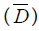. In this study, the mean dose was increased by 5% in the first treatment plan (all the organs included in the same ROI) and 5% in the second plan (organs treated as different ROIs). Equation (2) is valid only for uniform dose distributions and it gives an approximate solution to the change in response after a small change in dose.

In order to obtain a more accurate quantification of the change in response, one should preferably calculate the γ-value as a function of dose D or P(D). Assuming Poisson statistics, the response probability for uniform dose is: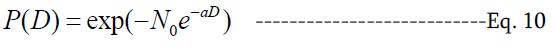so that the normalized dose response gradient becomes: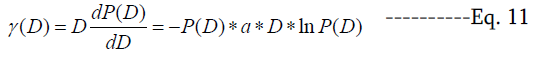Since Equation (10) gives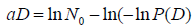and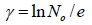, the normalized dose-response gradient for non-uniform doses is thus approximately given as: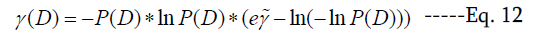The relative change in response as a function of the relative change in dose can thus be approximated as: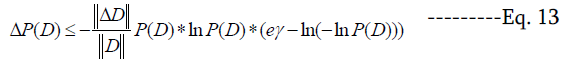or alternatively, if the mean dose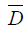is known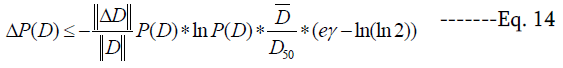In many circumstances, Equation (12) can be further approximated as: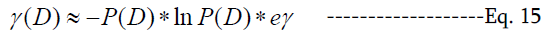where, exact equality prevails when P(D)=e-1. Hence, the approximate relative change in response as a function of the relative change in dose (Equation (13)) becomes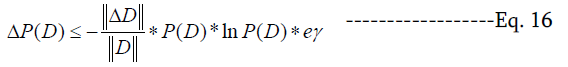After the accuracy validation of the Generalized Gamma value calculations with RayStation, an evaluation of practical merits of Generalized Gamma was performed. For this purpose, the functionality of the concept was studied for the case of a target volume consisting of two compartments. Firstly, the target was segregated into 2 compartments receiving doses, (D-ε) and (D+ε), where ε is the dose variance, and the corresponding responses are denoted as P1 and P2, respectively. So, according to Equation (16) and assuming that the responses are governed by Poisson statistics, the Generalized Gamma will be: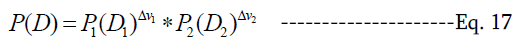where,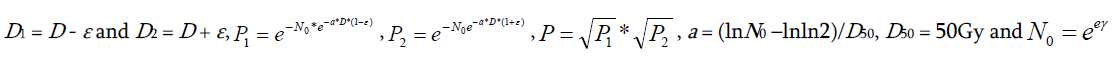Based on those formulas, generalized gamma is given by the following expression: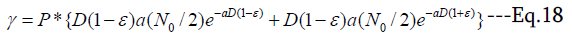The gradient of the dose-response function is also given by: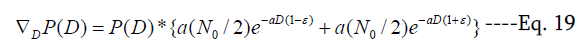In order to calculate the response of both compartments after a small increase in dose, (ΔD or δ) to get the theoretically estimated value for the change in response ΔP=P(DD)-P(D) the following formulations were used in Equation (17):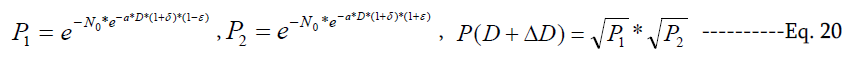In this study, we used ΔPreal=P(D+δ)-P(D) to represent the real value of the change in response. An approximate value for ΔP was calculated using the Equation (2 and 19). Having calculated both ΔPreal and ΔPapprox., the quantity (ΔPapprox.- ΔP real)/ΔPreal was plotted as a function of the prescribed dose D.

Finally, the above procedure was repeated assuming that the responses of the tissues are governed by normal distribution statistics. For this investigation, the normal cumulative distribution was employed for specified mean value μ and standard deviation σ values. In this study, the mean and standard deviation values were set equal to D50 = 50 Gy and σ = D50/γ(2π)1/2. The cumulative distribution returns a sigmoidal function for TCP and NTCP.

Results and Discussions

Taking into account the above basic definitions and formulas, as well as the calculations and the data from the RayStation treatment planning system, the theoretical behavior and merits of the generalized dose-response gradient were investigated. Additionally, the influence of the organ architecture (parallel-serial) and the usefulness of the generalized dose-response gradient in practical radiobiological treatment planning by using the RayStation platform were also studied.

Verification of RayStation computations
First, a verification of the calculations of RayStation regarding the values of the TCP and NTCP quantities was performed using an independent homemade software. At first, both the target and the normal tissue were assumed to belong to the same ROI, whose volume was characterized by the same radiobiological parameters (Table 1) and for this reason they both received the same uniform dose. Based on the calculations shown in the Appendix, the results for the TCP, NTCP and Generalized Gammas are presented in Table 2.

TABLE 1: Radiobiological and physical parameters used in the calculation of the TCP and NTCP measures.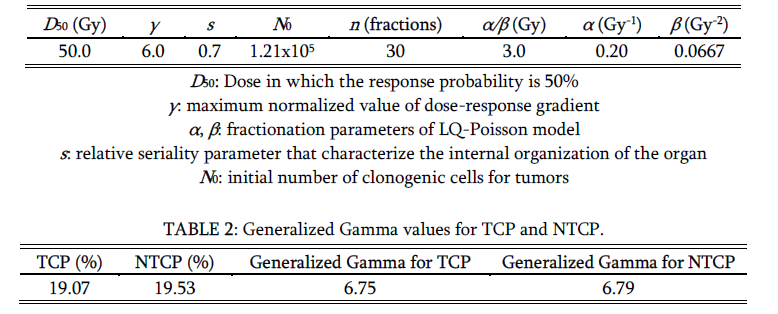As it can be noticed in Table 2, the values of TCP and NTCP are almost identical, which means that the calculations of RayStation were verified properly. The minor differences that appear between TCP and NTCP as well as the respective Generalized Gamma values stem from the fact that RayStation calculates the value of the objective function P+ using the expression P+=PB(1-PI) instead of P+=PB-PI that was used by the independent software. The determination of the optimal solution corresponds to the determination of the maximum P+ value. At this point, the value of PB is not equal to that of PI but to the derivative of P+.

TABLE 3: Summary of the results of Plan 1, which was optimized using a single ROI and D50 value for the target and bladder. However, the rest of the radiobiological parameters of two organs were different.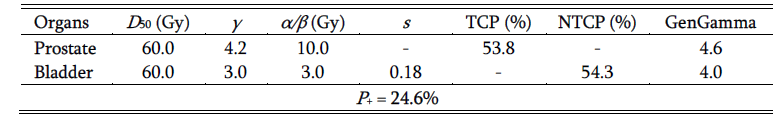Subsequently, further experimental treatment plans were produced in order to examine the behavior of the objective function P+, as well as the value of Generalized Gamma and in this way the effectiveness of the system. Based on the fact that both organs belong to the same ROI, we expect a small difference in the Generalized Gamma values due to the differences in γ and α/β values of the target and bladder. Also, the Generalized Gamma values are a little different due to the different response probabilities and parameter values. According to our results (Table 3), P+ is not zero, which indicates that the expression used in RayStation for P+ calculation is P+ = PB (1-PI).

Theoretical and experimental approach of Generalized Gamma
In Table 4, the results of another case in which the organs at risk belong to the same ROI as the target, are shown. In this case, however, there are two OARs and different radiobiological parameters characterize the target and the two OARs. Furthermore, different physical constraints were imposed during the development of the two plans (e.g. the minimum dose to the target was set to 60 Gy in Plan 3). In Table 4 it is noticed that the NTCP and the generalized gamma have the same value for both OARs, something that was expected because they receive exactly the same dose and they have the same radiobiological parameters.

In Table 5, the RayStation results for the case that the OARs belong to a ROI that is different than that of the target are shown. Those results are derived from three treatment plans, which were optimized using different combinations or organs involved (prostate, bladder and rectum), different radiobiological parameters and different physical constraints (e.g. the minimum dose to the target was set to 60 Gy in Plan 3). It can be seen that when the prescription dose constraint changed, the response of rectum was affected much more than those of the target and bladder and this is also reflected on the Generalized Gamma values.

TABLE 4: Summary of the results of two treatment plans, which were optimized using a single ROI for the target and OARs but different combinations of radiobiological and physical constraints (e.g. minimum dose to the target).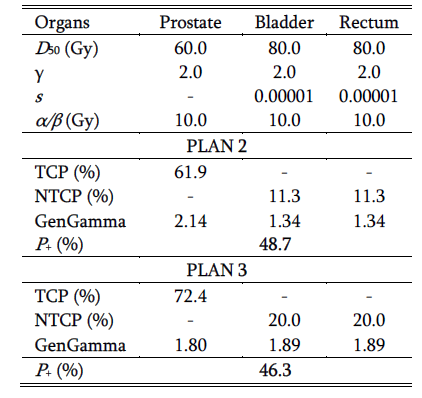TABLE 5: Summary of the results of three treatment plans, which were optimized using different combinations or organs involved (prostate, bladder and rectum) and different physical parameters (e.g. minimum dose to the target).After specifying the experimental treatment plans at RayStation and extracting the respective theoretical Generalized Gamma values, we tried to associate the experimental results for change in response, ΔP, with the theoretical formulae given in Equation (2, 14 and 16). In Tables 6-10, the results for theoretical and experimental changes in response probability (ΔPtheor. and ΔPexp.) for each case and corresponding treatment plan, are presented.

For the scenario of the organs within the same ROI (Plan 2 of Table 4), a set of values for a 5% increase in mean dose was acquired. Based on the results shown in Table 6, the experimental value of ΔP is closer to that of Equation (2). As a result, the experimental value can be used in Equation (2) to calculate the generalized gamma after the increase in dose. Table 6 shows that the values of the calculated generalized gamma of the different organs differ, which stems from the fact that the ΔP values are not identical.

TABLE 6: Comparison of the ΔPexp. and ΔPtheor. values for a 5% increase in dose. The Generalized Gamma values before and after the change in dose are also shown. These results stem from Plan 2 of Table 4.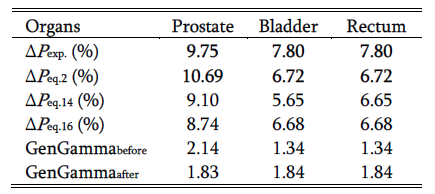The same procedure was repeated for the case that the target organ and the OARs belong to different ROIs (Plan 1 of Table 5) and the results are presented in Tables 7. According to those results it can be concluded that a 5% change in dose results in a change in response, which is close to that derived by Equation (16). This means that the γ(D) can be calculated using the expression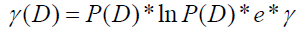.

TABLE 7: Comparison of the ΔPexp. and ΔPtheor. values for a 5% increase in dose. The Generalized Gamma values before and after the change in dose are also shown. These results stem from Plan 1 of Table 5.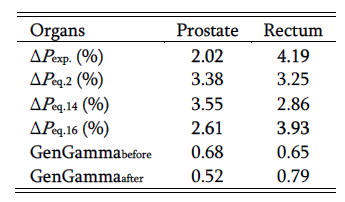Calculating the ΔPtheor. for the case that the target organ and the OARs belong to different ROIs, and applying physics dose constraints (Plan 2 of Table 5), it is noticed that for 5% change in dose the change in response, ΔP is close to that given by Equation (16). The respective results for ΔP and Generalized Gamma are presented in Table 8.

TABLE 8: Comparison of the ΔPexp. and ΔPtheor. values for a 5% increase in dose. The Generalized Gamma values before and after the change in dose are also shown. These results stem from Plan 2 of Table 5.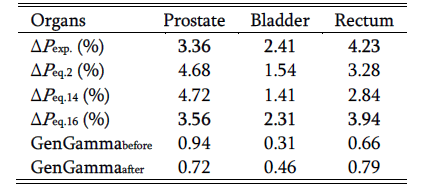According to the results shown in Tables 4-8 the theoretical value of response change, ΔP is in good agreement with the value returned by RayStation providing a verification of the validity of Equation (16).

The last part of this study concerns the association of the values of different quantities between RayStation and their theoretical calculation regarding the behavior of the change in response, ΔP and the Generalized Gamma for the D50 point of the dose-response relation. For this purpose, two treatment plans were developed by optimizing them so that the corresponding TCP and a NTCP values are almost 50%. In these cases, the behavior of ΔP and that of Generalized Gamma are shown in Tables 9 and 10, respectively. This was done by performing the previously described process, which involves the calculation of ΔPtheor. and the comparison with the respective ΔPexp. value.

As it can be noticed from Tables 9 and 10, the ΔPexp. value is in quite good agreement with the ΔPtheor. given by Equation (2) in both cases. This has resulted in the respective Generalized Gamma values to be almost the same as the initial values before the increase. In Table 10, the Generalized Gamma values before and after the change in dose for both plans are presented both as calculated by RayStation as well as theoretically.

TABLE 9: Summary of the results of three treatment plans, which were optimized using different combinations or organs involved (prostate, bladder and anterior rectum) and different physical parameters. Comparison of ΔPtheor. to ΔPexp. for 5% increase in dose. Upper panel: TCP 50%, Lower panel: NTCP50%.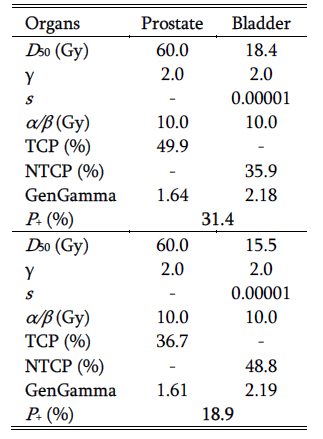After verifying the experimental with the theoretical value of Generalized Gamma for the scenario of increasing the mean dose by 5%, it was noticed that the experimental value of Generalized Gamma after the dose increase remains almost the same as the initial one. This is quite important for the verification of the accuracy of the TPS and its calculations because by taking into account the D50 and its properties, the behavior of the Generalized Gamma was shown to be the expected one.

TABLE 10: Comparison of ΔPtheor. to ΔPexp. for 5% increase in dose. Upper panel: TCP 50%, Lower panel: NTCP 50%.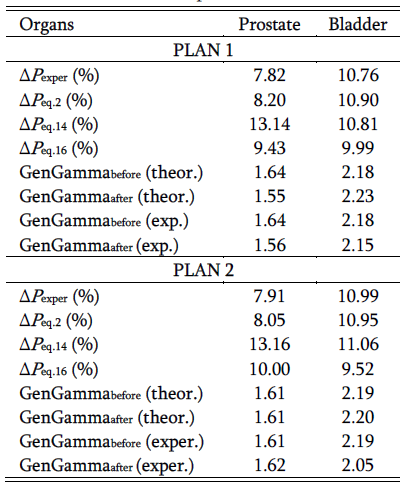Relative error of ΔPapprox. for target volume consisting of two compartments
Before examining the case of bifurcation of target volume into two compartments and the dependence of ΔPapprox. on the received dose, the behavior of Generalized Gamma was studied as a function of ε. As shown in Figure 1, we can notice that the generalized gamma decreases as the value of ε increases.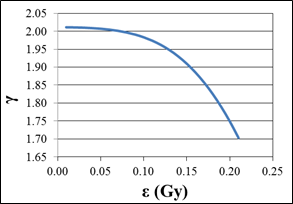FIG. 1:
The Generalized Gamma as a function of the dose change, ε.

Figure 1 could be quite comparable with the findings of the study of Lind et al.17, where γ had been computed for the case of having different number of clonogens for the two compartments as shown in Figure 2.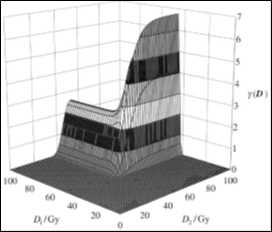FIG. 2: γ(D) for the two compartments of the target receiving doses D1 and D2 and having N01 and N02 number of clonogens.

Furthermore, the relative error ΔPapprox as a function of dose has been studied and plotted (Figure 3) for the case of the target volume consisting of two compartments with N1 and N2 (=N0) number of clonogens receiving doses D1 and D2, respectively. More specifically, the Poisson model was used to calculate the response P(D) for the two compartments with doses (D+ε) and (D-ε) and the response P(DD) for increasing the dose at D by ΔD. In addition, the values of ΔPreal=P(DD)-P(D) and ΔPapprox.D/D*gamma were calculated and their relative deviation as a function of the prescribed dose was plotted. The result is presented in Figure 3, in which ε = 0 and δ = 1%.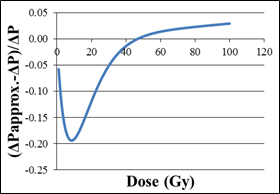FIG. 3: Relative error of ΔPapprox as function of dose, for ε=0 and δ= 1%

In Figure 3, it can be noticed that the point at which the best agreement between ΔPreal and ΔPapprox. occurs is the D37 point. This point corresponds to a 37% response and the relative difference is 9.76*10-4. That makes sense considering the behavior of the Poisson model at D37.

Another model was also used for calculating the response, P in order to plot the relative error of ΔPapprox, expecting more precise results and smoother curve.  In this case, the response P is represented by the normal cumulative distribution function, which is computed in terms of a special function called Probit function (error function), for specific mean value and standard deviation. In this analysis, the mean value μ = 50Gy and the standard deviation is σ=D50/(γ*(2π)1/2). The expectation from this calculation is the sigmoidal curve that represents the response function. For the computation of ΔPapprox, the calculation of generalized gamma was not needed, due to the fact that the gradient of P is a cumulative density function (CDF) and tends towards a probability density function (PDF). Figure 4 shows the behavior of the relative deviation ΔPapprox as a function of dose, using the Probit (error) function.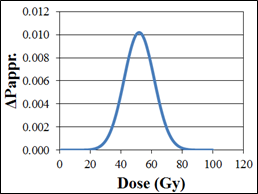FIG. 4: ΔPapprox as a result of the probit function.

Even with the Gaussian-shaped curve, it can be noticed that the maximum value of the curve occurs at a dose slightly higher than 50Gy. Despite that fact, we notice from the following plot (Figure 5) that the best approximation between ΔPreal and ΔPapprox. appears at D50, which is 4.34*10-4. From Figure 5 it can be easily concluded that the probit function gives a smoother curve than the Poisson model and provides a much better agreement between the ΔPreal and ΔPapprox. quantities.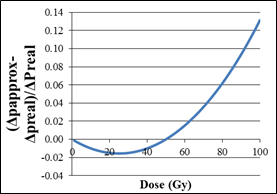FIG. 5: The relative deviation of ΔP using probit function, for ε = 0 and δ = 1%.

The results of this study are strongly dependent on the accuracy of the radiobiological models and the parameters describing the dose-response relation of the different tumors and normal tissues. This accuracy depends on many factors such as the mathematical formalism of each model, the assumptions on which it is based, the biological mechanisms that it accounts for etc. In this way, certain models describe better the data from certain clinical conditions (e.g. cancer type, treatment protocol) than other models and the opposite happens in other cases. Furthermore, the determination of the model parameters expressing the effective radiosensitivity of the tissues is subject to uncertainties imposed by the inaccuracies in the patient setup during radiotherapy, lack of knowledge of the inter-patient and intra-patient radiosensitivity and inconsistencies in treatment methodology. Consequently, the determined model parameters (such as the D50, γ and s) and the corresponding dose-response curves are characterized by confidence intervals.

In the present study, most of the tissue response parameters have been taken from recently published clinical studies, where these parameter confidence intervals have been reduced significantly. In this study, due to the fact that many parameters affect the calculation of the TCP/NTCP values (such as the clinical endpoints of the different tissues, the treatment protocol etc.) it would not be possible to provide any global error bars to those values. This could be possible only if the clinical problem examined was reduced to a very specific clinical situation where a TCP uncertainty of about 5% is usually expected. However, due to the comparative nature of the study, this uncertainty in the absolute knowledge of the expected responses does not affect the general conclusions of this investigation.

Conclusion

The present analysis indicates that the concept of Generalized Gamma is very important for the optimization of a treatment plan because it is a factor that can predict the organ which will most likely be affected after a change in dose. That is because the highest change in response is observed for the organ with the highest Generalized Gamma. Irrespective of which organ has the highest response, in a given clinical case the highest change in response after a small change in dose cannot be predicted with certainty because usually the organ with the highest TCP or NTCP tend to have the highest change in response after a small increase in dose. In the scenario of a small change in dose, the organ with the highest Generalized Gamma factor is most likely the organ that is affected the most. Another significant finding to be highlighted is the agreement between the theoretically calculated values of TCP, NTCP, P+ and Generalized Gamma with those from the RayStation treatment planning system.

Conflict of Interest

The authors declare that they have no conflicts of interest. The authors alone are responsible for the content and writing of the paper.

Conflict of Interest

Generalized Gamma calculations for TCP and NTCP
Using Equation (5), the following formula for voxel response can be derived: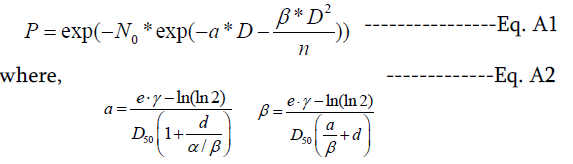d =D/n is the dose per fraction and n is the number of fractions. α and β are the fractionation parameters of the linear-quadratic (LQ) model and account for the early and late effects expected.22-24 N0 is the initial number of the clonogenic cells for tumors or the initial number of functional subunits for healthy tissues. Parameters α and β are specific for every organ and specific for the kind of injury (endpoint) considered and can be calculated only from clinical data.

Based on Equation (A1, A2 and 7), the TCP, NTCP and corresponding generalized gamma values for the five bin DVH shown in Table A1 are calculated by the following equations: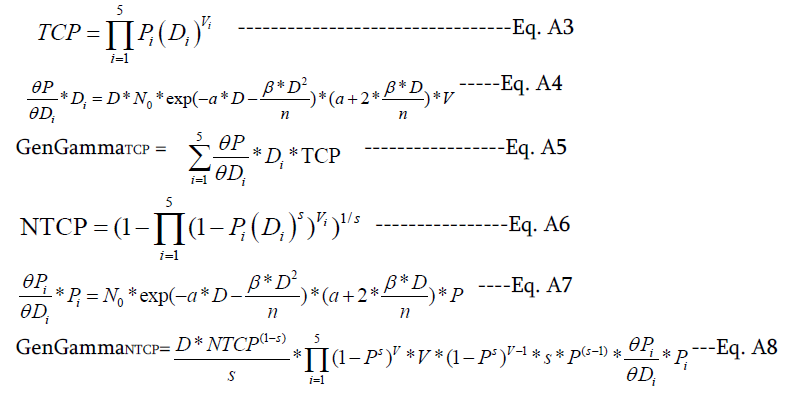TABLE A1. Radiobiological parameters and calculations of the generalized gamma for a tumor and a healthy tissue, respectively.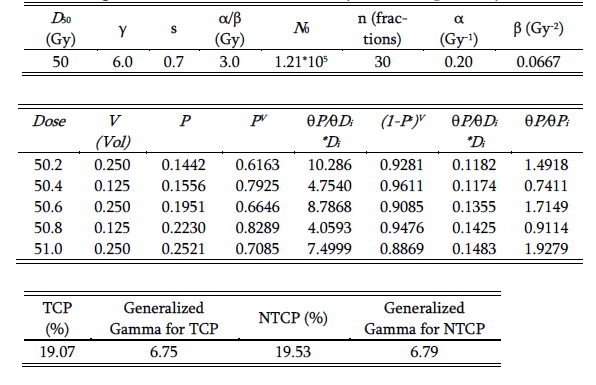Received September 29, 2014; Revised November 19, 2014; Accepted November 21, 2014; Published Online December 02, 2014

Corresponding author: Panayiotis Mavroidis; Department of Radiation Oncology, University of Texas Health Science Center, San Antonio, TX, USA.